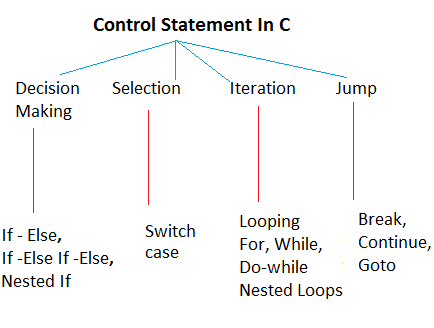Control Statements In C

As the name suggest control statements controls over the execution of programming flow. Let’s understand different types of control statements.Types Of Control Statement In C. ByTechAchievers.com

Decision Making Statement

Decision Making Statements help programmer to write conditional based coding. If and Else are the decision making keywords.Execute some piece of code if condition fails then execute other code.The “if”part works on condition’s true part and “else” works on otherwise part of condition. If and Else blocks are enclosed within the { } (curly braces).
``````
Example:

``````if (condition) {

Condition satisfy: write code here

}

else {

Condition not satisfy: write code here

}``````
Note:
If is there only one line statement to be executed within the if or else block then we can avoid {} (curly braces)
``````
Example:

``````if (condition)

Condition satisfies: write code here

else

Condition does not satisfy: write code here``````

``````

Multiple If - Else Statement

This is the case when we have multiple conditions, if one condition does not satisfy then check with other condition and so on. If all conditions are not going to satisfy then execute else part.
``````
Example:

``````if (condition1) {

Condition1 satisfies: write code here

}

else if (condition2) {

Condition2 satisfies: write code here

}

else if (condition3) {

Condition3 satisfies: write code here

}

else {

Condition does not satisfy: write code here

}

``````
Nested If - Else Statement

This is the case when we have condition within the condition.We may have condition check inside the if, else or both blocks. Nested condition will work same as other conditions we can apply multiple if as well.
``````
Example:

``````if (condition1) {

Check another condition

if (condition2) {

Condition satisfy: write code here

}

else  {

Condition not satisfy: write code here

}

}

else  {

Condition not satisfy: write code here

}
``````
Use Of Conditional Operators

We have learned different types of decision making statements, we have seen only one condition with if - else in the above examples. When we write any condition we have to use conditional operators.

Ex: assignment,comparison,relational,logical conditions etc. Operators In C

Here I am discussing about relational and ternary operators.We may have multiple conditions for example: perform the task if number is > 2 but less < 10. To combine multiple conditions C provides some relational operators.

&& ( And Operator ) -

And operator combines multiple conditions and all conditions should be satisfied, only in this case if block will execute.
``````
Example:

``````if ( condition1 && condition2 && condition3 ) {

// execute if all conditions are satisfy

}``````

``````
|| ( Or Operator ) -

Or operator combines multiple conditions if them satisfies if block will execute.
``````
Example:

``````if ( condition1 || condition2 || condition3 ) {

// execute if any one condition is satisfy

}``````
``````
! ( Not Operator ) -

Not operator works with negative condition for example: if x value is not equal to 10 then do some action etc.
``````
Example:
``````
if ( x!=10 ) {

// execute if x is not equals to 10

}

if ( x!10 ) - This is invalid condition``````

``````
Ternary Operator ( ? : ) -

Ternary operator provides simple and easy way to check one line conditions. It used with assignment operators ( = ) that means the result of condition stores into a variable.

? returns true part of condition.

: returns false part of condition
``````
Example:

``````int a = ( x > 10 ? 3 :7 );

If x >7 true then ? returns true part value is 3 to a variable

if condition not satisfy then : returns false part value is 7
to a variable.``````

``````
Simple If - Else Example In C

Write a program to ask any number from user and find it is even or odd number.
``````
````#include <stdio.h>````

````void main()```` {

int number;

printf("Enter Any Number");

scanf("%d",&number);

if ( number%2 == 0) {

printf("\n Given number is even number");

}

else {

printf("\n Given number is odd number");

}

}

``````
Note: % operator gives reminder of the number. Every even number reminder is 0 when it divides by 2.

Multiple If - Else Example In C

Enter Write a program to calculate area of circle, square and rectangle.Enter your choice – ‘S’ for square, ‘C’ for Circle and ‘R’ for Rectangle. Choice should not be case sensitive.
``````
#include <stdio.h>``````

````void main()```` {

char choice;

int length,width,side;

scanf("%c",&choice);

if ( choice =='s' || choice == 'S') {

printf("\nEnter side of square");

scanf("%d",&side);

area = side*side;

}

else if ( choice =='c' || choice == 'C') {

printf("\nEnter radius of circle");

}

else if ( choice =='r' || choice == 'R') {

printf("\nEnter length and width of rectangle");

scanf("%d%d",&length,&width);

area = length*width;

}

printf("\nArea is - %f",area);

}

``````
Note: Lower case and upper case characters behave differently so that need to check both cases.

Nested If - Else Example In C

Write a program for Bank customer. Enter customer's name account number , amount and action to be performed.

If,

Action =1 , deposit amount.

Action =2 , withdrawal amount.

Action =3 , display balance amount.

``````
````#include <stdio.h>````

````void main()```` {

char customer_name;

char account_number;

int balance , action , deposite_amount ,withdrow_amount ;

fgets(customer_name,100,stdin);

fgets(account_number,100,stdin);

scanf("%d",&balance);

scanf("%d",&action);

if (action == 1) {

printf("\nWow! You Selected Deposite Action");

printf("\nPlease Enter Deposite Amount");

scanf("%d",&deposite_amount);

balance = balance + deposite_amount;

}

else if (action ==2) {

printf("\nWow! You Selected Withdow Action");

printf("\nPlease Enter Withdrow Amount");

scanf("%d",&withdrow_amount);

balance = balance - withdrow_amount;

}

else{

printf("\nWow! You Selected Balance Action");

}

printf("\nYour balance  amount -%d",balance);

}

``````
Ternary Operators Example In C

Write a program to enter two numbers calculation choice from user.
if operation = 1 then add number , otherwise subtract numbers.
``````
``````#include <stdio.h>
``````
````void main() ````{

int num1,num2,operation,result;

printf("Enter 1st number");

scanf("%d",&num1);

printf("Enter 2nd number");

scanf("%d",&num2);

printf("Enter operation");

scanf("%d",&operation);

result = (operation==1 ? num1+num2 : num1-num2);

printf("Result is %d",result);

}
``````

Thanks for reading, please do share and comment your suggestions.